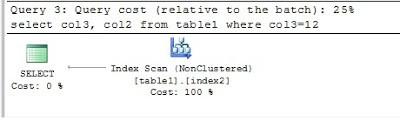Select Page

SQL Server : How to decide which composite index to create?

Creating new indexes can be a significant change and it is important to note that adding unnecessary indexes is not a good strategy. While indexes lead to better READ performance they will reduce the WRITE performance on the tables.

If you have more than one SELECT query to tune and create indexes for then you have to analyze if there is one index that will satisfy all the queries. If one index won’t suffice for all the queries then find the least number of indexes and their definitions that will improve the performance of those queries.

To illustrate an example, I am going to use the following table

`CREATE TABLE [dbo].[table1](`

[col1] [int] NULL,

[col2] [int] NULL,

[col3] [int] NULL,

[col4] [int] NULL

`) ON [PRIMARY]`

Consider the following SELECT statements and lets see what kind of indexes will help.

1) select col1, col2 from table1 where col1=10

2) select col1, col2, col3 from table1 where col1=10 and col3=3

3) select col3, col2 from table1 where col3=12

4) select col1, col2, col3, col4 from table1 where col1=10 and col3=12 and col4=15

Note: Any column that is part of the selected columns but is not part of the conditions in the WHERE clause, can be part of the included columns of the index. That column does not need to be part of the columns on which the index is created. In all the above queries col2 is part of the selected columns but not part of the WHERE clause.

For the first query all you need is an index on col1 that also includes col2. This will result in index seeks.

`CREATE NONCLUSTERED INDEX [index1] ON [dbo].[table1]`
`( [col1] ASC )INCLUDE( [col2])`

For the second query you will need an index on col1 and col3. Also include col2 in that index.

`CREATE NONCLUSTERED INDEX [index2] ON [dbo].[table1]`
`( [col1] ASC, [col3] ASC ) INCLUDE( [col2])`

Note that since the first column in index2 is col1, it will lead to index seeks for the first query. Hence, index2 will make index1 redundant.

But for the third query the index ‘index2’ will not help. This query will lead to index scans. The order of the columns in a composite index is important. In index2, col3 is not the first column in the order hence it will lead to index scans for any query which has a WHERE clause on just the col3.So the following index would be appropriate. This index will be created on col3 and include col2.

`CREATE NONCLUSTERED INDEX [index3] ON [dbo].[table1]`
`( [col3] ASC ) INCLUDE( [col2])`

Now consider the 4th query, to force an index seek you will have to create the following index.

`CREATE NONCLUSTERED INDEX [index4] ON [dbo].[table1]`
`( [col1] ASC, [col3] ASC, [col4] ASC ) INCLUDE( [col2])`

Notice that index4 will lead to index seeks for query 1 and 2. So there is no need create index1 and index2

If you analyze the indexes closely you will see that Index4 will be sufficient for Queries 1, 2 and 4.

To force index seeks for all the queries mentioned above Index4 and Index 3 will be sufficient.